Multiply and Match – Multiplication Activity – Multiply by 2, 3, 4, 5, 6, 7, 8 and 9

Cool Math Activity – Multiply by 2, 3, 4, 5, 6, 7, 8 and 9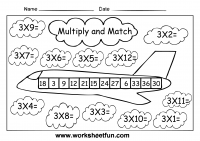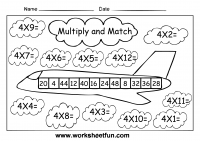Multiplication Worksheets

Multiplication

Multiplication – Basic Facts

Multiplication – Cubes

Multiplication – Horizontal

Multiplication – Quiz

Multiplication – Test

Multiplication – Vertical

Multiplication Target Circles

Multiplication Word Problems

Multiplication-1 Digit

Multiplication-2 Digit by 2 Digit

Multiplication-3 Digit by 1 Digit

Multiplication-3 Digit by 2 Digit

### New Worksheets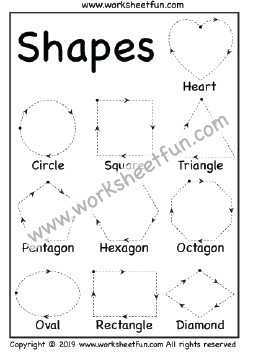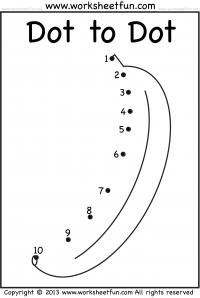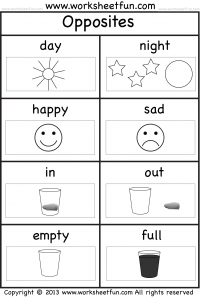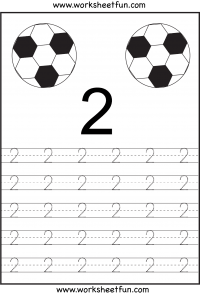### Most Popular Preschool and Kindergarten Worksheets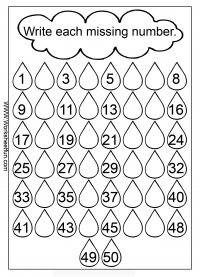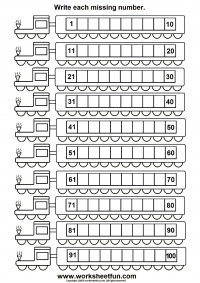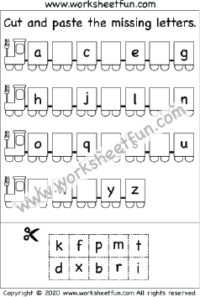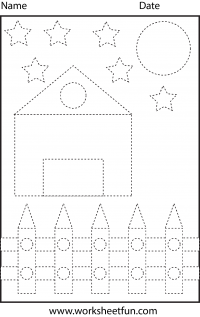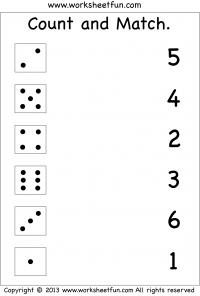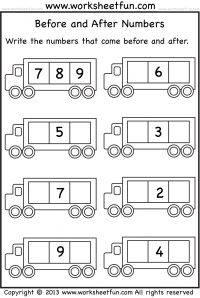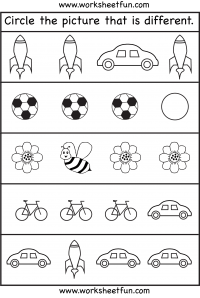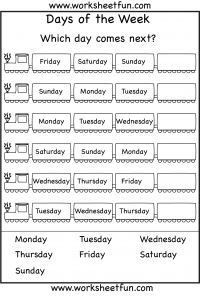#### Kindergarten Worksheets